Options
All
• Public
• Public/Protected
• All

#This project is part of the @thi.ng/umbrella monorepo.

nD cubic & quadratic curve analysis, conversion, interpolation, splitting.

Current implementations partially based on toxiclibs (Java) and Clojure version of thi.ng/geom.

### Status

STABLE - used in production

Search or submit any issues for this package

## Installation

``````yarn add @thi.ng/geom-splines
``````
``````// ES module
<script type="module" src="https://unpkg.com/@thi.ng/geom-splines?module" crossorigin></script>

// UMD
<script src="https://unpkg.com/@thi.ng/geom-splines/lib/index.umd.js" crossorigin></script>
``````

Package sizes (gzipped, pre-treeshake): ESM: 2.18 KB / CJS: 2.30 KB / UMD: 2.28 KB

## Usage examples

Several demos in this repo's /examples directory are using this package.

A selection:

ScreenshotDescriptionLive demoSource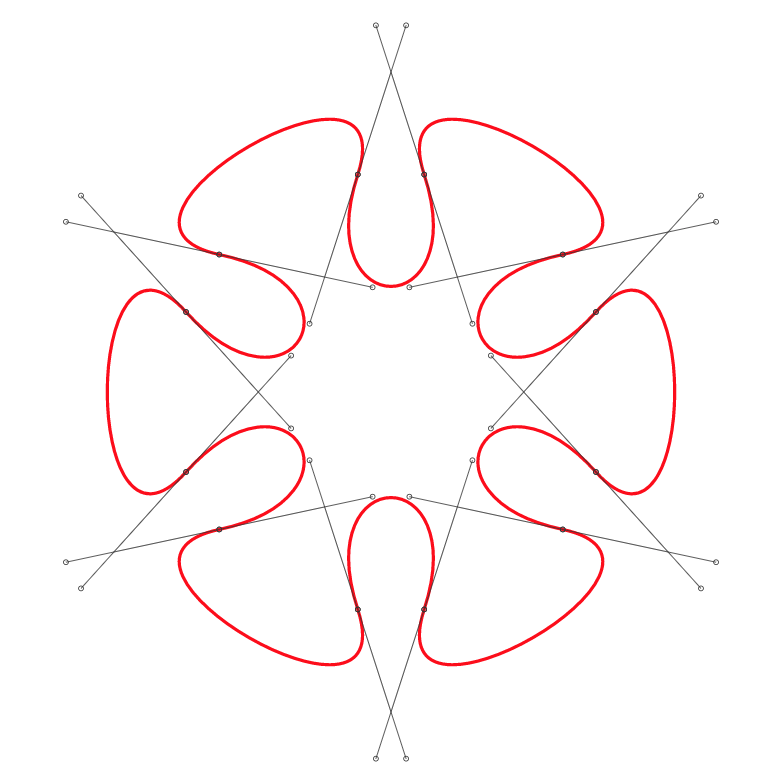Polygon to cubic curve conversion & visualizationDemoSource

## API

Generated API docs

### Cubic curve conversion from polygons & polylines

Currently, there're two ways to convert a polygon/polyline into a sequence of cubic curve segments. Both modes support customizable curve tightness.

Furthermore, both conversion modes can be instructed to use uniformly scaled control point tangents: In uniform mode, the tangents have a uniform, user defined length, resulting in equidistant control points for each poly vertex. In non-uniform mode, each tangent is scaled by the length of its parent poly edge.

#### Poly vertices as control points

In this mode the curve always goes through the midpoints each polygon edge, with the original polygon vertices being used to compute control points.

Proportional tangent scaleUniform tangent scale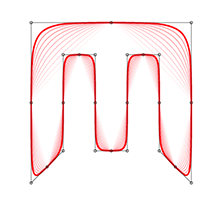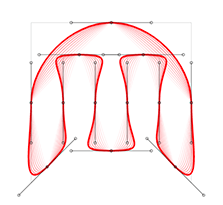#### Poly vertices as break points

In this mode the curve always goes through the original polygon vertices and additional control points are created via symmetric tangents at each poly vertex. The tangents themselves are computed via the bisector of each vertex corner, taking into the convexity of each poly vertex.

Proportional tangent scaleUniform tangent scale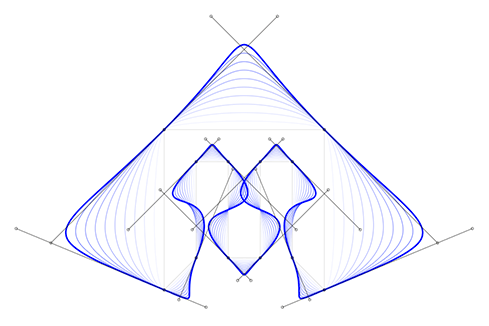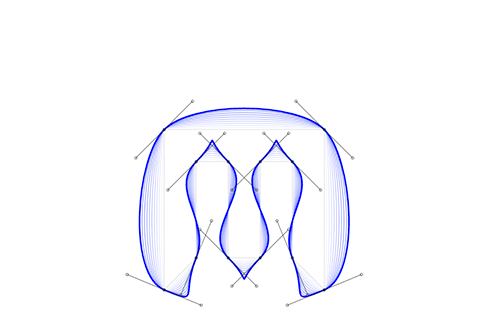## Authors

Karsten Schmidt

If this project contributes to an academic publication, please cite it as:

``````@misc{thing-geom-splines,
title = "@thi.ng/geom-splines",
author = "Karsten Schmidt",
note = "https://thi.ng/geom-splines",
year = 2016
}
``````